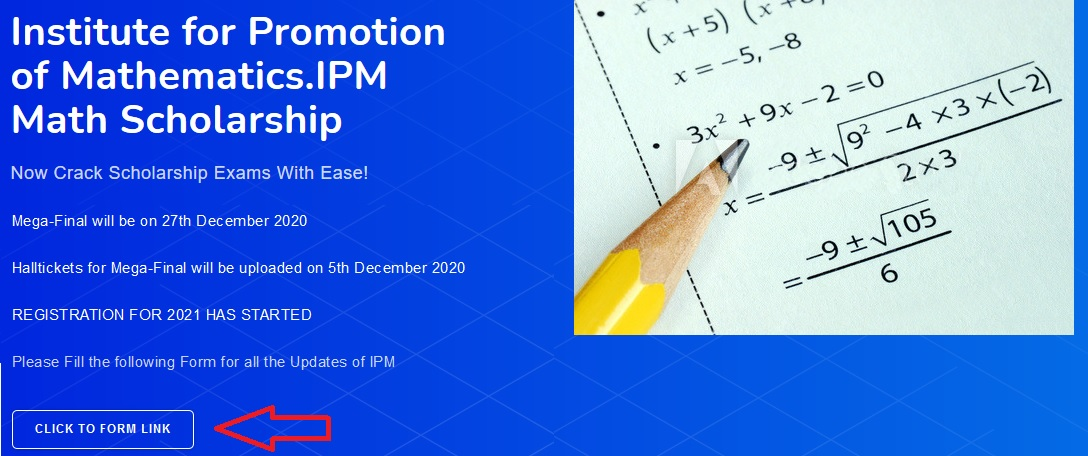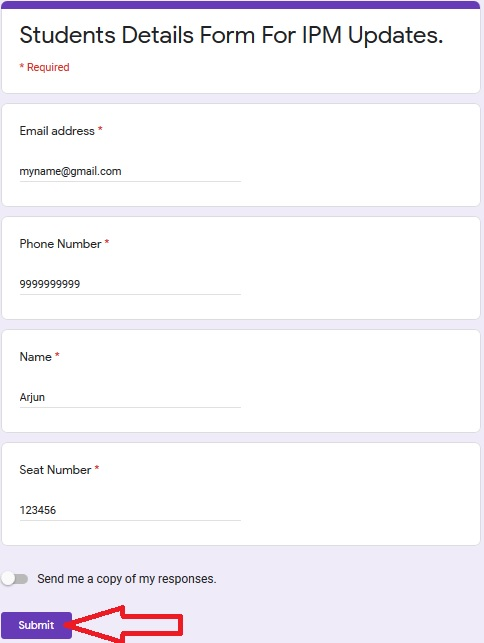You are here: Home > Scholarship Exam/Test
All posts from

# IPM Math Scholarship 2021 Institute for Promotion of Mathematics : ipmmathscholarship.com

Organisation : Institute for Promotion of Mathematics IPM
Scholarship Name : IPM Math Scholarship 2021
Applicable For : Student studying in Stds.II to IX
Exam Dates : 27th December 2020
Website : https://www.ipmmathscholarship.com/

## IPM Math Scholarship

Institute for Promotion of Mathematics.IPM Math Scholarship. Now Crack Scholarship Exams With Ease! Institute for Promotion of Mathematics is a unique Institute working since 1996 for school going children.

This is a competitive Examination for various standards where the mathematical skills, mental ability, calculation speed, critical analysis and accuracy of a child & application of short-cut methods are tested.

## Who is Eligible?

Any student studying in Stds.II to IX of any Recognised school with S.S.C, I.C.S.E or C.B.S.E & I.B or any other Board pattern is eligible to appear for this exam

## How to Apply?

Just follow the below steps to apply for IPM Math Scholarship 2021.

Steps :
Step 1 : Visit the official website through provided above.
Step 2 : Read the instructions carefully and click on “Click To Form Link”.Step 3 : Fill the Application Form with the required details
Step 4 : Finally click on “Submit” button to complete your registration.## Nature of Exam

The students appearing for this exam will be tested in Mathematics only.The question paper will consists of 40 questions, of 2.5 marks each. The entire paper will be objective in nature The duration of the examination will be 60 minutes for final examination. Students are requested to study the OMR answer sheet available on this site

We conduct 2 exams every year. Final exam is held in Arpil and those who secure more than certian marks in that exam are eligible to appear for Level 2 exam also called as Mega final which typically held in Aug .

## Study Material

We have 3 books for each standard. 1 text books , 1 book of past question papers and 1 book for solved past question papers. Besides there are lot of online tests available as well

## Important Dates

** Mega-Final will be on 27th December 2020
** Hall tickets for Mega-Final will be uploaded on 5th December 2020

## Syllabus

Standard II :
** Numbers
** Multiplication
** Fractions
** Money
** Time and Calendar
** Shapes
** Measurment
** Data Handling
** Analytical Thinking

Standard III :
** Numbers
** Roman Numberals
** Multiplication
** Division
** Measurement of Length
** Measurement of Mass
** Measurement of Capacity
** Money
** Time
** Calender Fun
** Fractions
** Pictorial Representation
** Geometry

Standard IV :
Numbers :
** Kind of Numbers
** Place value of the digits
** Comparison of Numbers and,
** Numbers between certain two no.
** Sequence and Series of Numbers
** Magic square
** Roman Numerals
** Square and square Numbers

Fundamental Operations :
** Multiplication and Division
** BODMAS

Equations :
** Use of letters in simple equations
** Algebric Expressions

General :
** Divisibility
** L.C.M. and H.C.F
** Common Fractions
** Decimal Fractions
** Decimal Units of Measurements
** Measurement of Time Calendar and Other Units of Measurement
** Units of Measurement of Articles

Geometry :
** Angle
** Triangle
** Circle

Standard V :
** Numbers
** Place value of the digits
** Sequence and Series
** Square Numbers
** Fundamental Operations (Addition and Subtraction)
** Fundamental Operations (Multiplication and Division)
** BODMAS
** Equations
** Algebraic Expressions
** LCM and GCD (HCF)
** Fractions
** Decimal Fractions
** Unitary Method
** Percentage
** Profit and Loss
** Specd
** Perimeters
** Area – (Triangle, Square and Rectangle)

Standard VI :
Algebra :
** Integers
** Square and Square Root
** Average
** Arithmetic Equations
** Sequence and Series
** Unitary Method & Time work Relation
** Simple & Compound Interest
** Ratio & Proportion

Geometry :
** Circle
** Perimeter & Area
** Volume & Surface Area
** Polygons
** Parallel Lines
** Graphs

Standard VII :
** Fractions
** Decimal Fraction
** Square and Square Roots
** Average
** Indices
** Ratio and Proportion
** L.C.M. and H.C.F.
** Percentage
** Profit and Loss
** Simple and Compound Interest.
** Time, Speed and Distance
** Time and Work
** Algebraic Expressions
** Simple Equations
** Angles
** Polygons
** Perimeter and Area of Different Solids
** Volume and Surface Area of Different Solids

Standard VIII :
** Set Theory
** Square Roots and Cube Roots
** Rational Numbers
** Indices (Exponents)
** Identities Expansion
** Identities Factors
** Linear Equations
** Simultaneous Equations
** Polynomials
** Compound Interest
** Statistics
** Congruence of Triangles
** Circle
** Volume and Surface Area

Standard IX :
Arithematic :
** Logarithms
** Ratio and Proportion

Algebra :
** Sets
** Surds
** Polynomials
** Factorization of Polynomials
** Rational Algebraic Expression
** Linear Equations in one unknown
1.